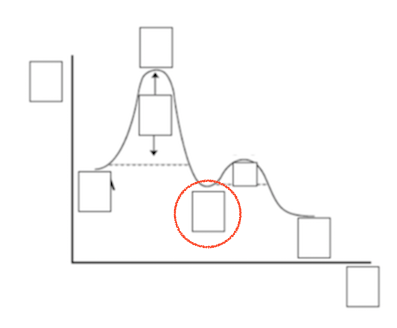# Problem: Label the multi-step reaction energy diagram below using the letters corresponding to the labels on the left. There are more labels than needed; each label can be used only once.A) Reaction intermediateB) Ea (step 2)C) ReactantsD) ProductsE) Transition stateF) Ea (step 1)G) CatalystH) EquilibriumI) kfJ) krK) Reaction coordinateL) Energy

###### FREE Expert Solution
96% (159 ratings)
###### Problem Details

Label the multi-step reaction energy diagram below using the letters corresponding to the labels on the left. There are more labels than needed; each label can be used only once.

A) Reaction intermediate

B) Ea (step 2)

C) Reactants

D) Products

E) Transition state

F) Ea (step 1)

G) Catalyst

H) Equilibrium

I) kf

J) kr

K) Reaction coordinate

L) EnergyWhat scientific concept do you need to know in order to solve this problem?

Our tutors have indicated that to solve this problem you will need to apply the Energy Diagram concept. If you need more Energy Diagram practice, you can also practice Energy Diagram practice problems.

What is the difficulty of this problem?

Our tutors rated the difficulty ofLabel the multi-step reaction energy diagram below using the...as medium difficulty.

How long does this problem take to solve?

Our expert Chemistry tutor, Jules took 3 minutes and 9 seconds to solve this problem. You can follow their steps in the video explanation above.

What professor is this problem relevant for?

Based on our data, we think this problem is relevant for Professor Pavel's class at UNC.